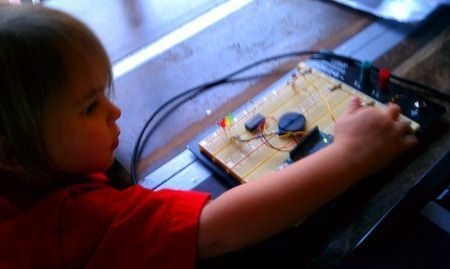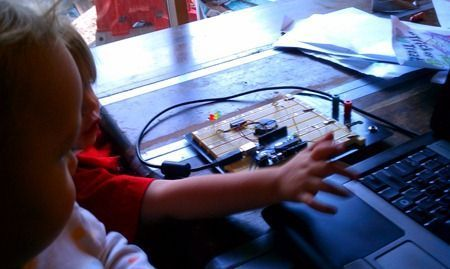Tip! This post is part of a series on my adventures with Arduino

Today’s build was a fun one for Thing 1. It’s basically just two CdS light sensors tied to a set of LEDs and a buzzer. If you cover either of the sensors (or both), a different tone plays and an LED lights. Like so:

#### Build#### Code

There’s nothing too fancy going on here. The only real trick (if you can call it that) is to read in the ambient analog values at startup so you can compare against them later. This is much more effective than hard-coding a threshold.

``````const int BuzzPin = 5;
const int BuzzDuration = 50;
const int Tones[] = { 800, 1000, 1200 };
const int LedPins[] = { 9, 10, 11 };
const int CdsPins[] = { 0, 1 };
int ActiveLed = -1;
int CdsSquelch;

void setup() {
Serial.begin(9600);

pinMode(LedPins, OUTPUT);
pinMode(LedPins, OUTPUT);
pinMode(LedPins, OUTPUT);
pinMode(BuzzPin, OUTPUT);

// read in the ambient light value to be used as a threshold
// this is helpful since the amount of ambient light changes all the time
for(int i = 0; i < 2; i++){
}
}

void loop() {
int Led;

// convert the CdS values into a boolean
// - true if the light detected is less than 90% of ambient
for(int i = 0; i < 2; i++){
}

// if both cells are covered...
Led = 1;
}
// if one cell is covered
Led = 0;
}
// if the other cell is covered
Led = 2;
}
// if neither cell is covered
else{
Led = -1;
}

// if something is covered, beep and blink
if(Led != -1){
Ding(Led);
digitalWrite(LedPins[Led], HIGH);
}

// turn off the previously lit LED if necessary
if(Led != ActiveLed){
digitalWrite(LedPins[ActiveLed], LOW);
ActiveLed = Led;
}
}

void Ding(int light){
// e.g. 1 / 2048Hz = 488uS, or 244uS high and 244uS low
// to create 50% duty cycle
// http://www.arduino.cc/cgi-bin/yabb2/YaBB.pl?num=1231194692
int Osc = 1000000 / Tones[light] / 4; // in microseconds

// compute the number of iterations needed to hold
// the nfote the desired duration
int Iterations = Tones[light] * ((float)200 / 1000);

// play tone
for (long i = 0; i < Iterations; i++ )
{
digitalWrite(BuzzPin, HIGH);
delayMicroseconds(Osc);
digitalWrite(BuzzPin, LOW);
delayMicroseconds(Osc);
}
}
``````